# Huyghen's principle and interference of light

## 4) Reflection of and Refraction of plane waves using Huygens�s principle

i) Reflection of plane wave at plane surface:-
• Consider the figure given below which shows incident and reflected wave fronts when a plane wave fronts travels towards a plane reflecting surface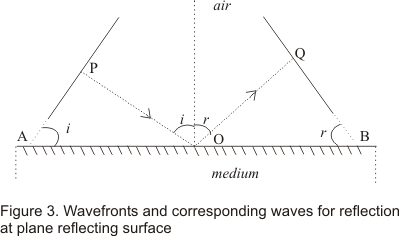• POQ is the ray normal to both incident and reflected wave fronts
• The angle of incidence i and angle of reflection r are the angles made by incidence and reflected rat respectively with the normal and these are also the angles between the wave fronts and the surface as shown in the figure 3
• The time taken by the ray POQ to travel from incident wave front to then reflected one is
Total time from P to Q= t=PO/v1 + OQ/v1
where v1 is the velocity of the wave. From figure (3)• There can be different rays normal to incident wave front and they can strike plane reflecting surface at different point O and hence they have different values of OA
• Since tome travel by each ray from incident wave front to reflected wave front must be same so, right side of equation (1) must be independent of OA.This conditions happens only if
(sini-sin r)=0
or i=r
Thus law of reflection states that angle of incidence i and angle of reflection are always equal
ii) Refraction of plane waves at plane surfaces:-
• Consider the figure given below which shows a plane surface AB separating medium 1 from medium 2
• v1 be the speed of light in medium 1 and v2 the speed of light in medium 2• Incident and refracted wave front makes angles i and r' with surface AB where r' is called angle of refraction
• Time taken by ray POQ to travel between incident and refracted wave fronts would be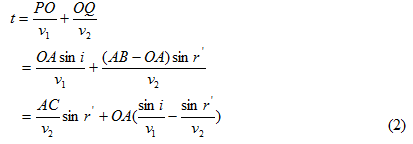• Now distance OA would be different for different rays .So time t should be independent of any ray we might consider
• This can be achieved only if coefficient of OA in equation (2) becomes equal to zero or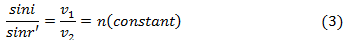• Equation (3) is nothing but Snell�s law of refraction where n is called the reflective index of second medium with respect to the first medium.

iii) Refractive index
• The ratio of phase velocity of light c in vacuum to its value v1 in a medium is called the refractive index n1 ( or μ1) of the medium .Thus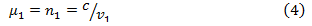• When light travels from medium 1 to medium 2,what we measure is the refractive index of medium 2 relative to medium 1 denoted by n12 ( or μ12).Thus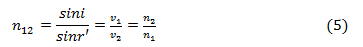where n1 is refractive index of medium 1 with respect to vacuum and n2 is refractive index of medium 2 w.r.t. vacuum
• When light travels from one medium to another the frequency ν=1/T remains same i.e. ν1=ν2
• Since the velocities of light v1 and v2 are different is different medium ,the wavelength λ1 and λ2 are also different i.e.,the wavelength of light in the medium is directly proportional to phase velocity and hence inversely proportional to the refractive index

## 5) Principle of Superposition of waves

• When two or more sets of waves travel through a medium and cross one another the effects produced by one are totally independent of the
• At any instant the resultant displacement of a particle in the medium depends on the phase difference between the waves and is the algebraic sum of the displacement it would have at the same instant due to each separate set. This is known as the principle of superposition of waves and forms the basis of whole theory of interference of waves discovered by Young in 1801

• If at any instant y1,y2,y3,--- are the displacements due to different waves present in the medium then according to superposition principle resultant displacement y at any instant would be equal to the vector sum of the displacements (y1,y2,y3) due to the individual waves i.e.,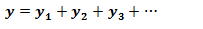• The resultant displacement of the particles of the medium depends on the amplitude ,phase difference and frequency of the superposing waves
• Consider two waves of same frequency f and wavelength λ travelling through a medium in the same direction and superpose at any instant of time say t
• Equation of these waves at time t is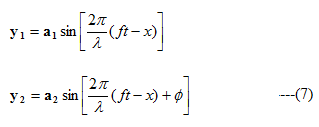where φ is the phase difference between the waves
• According to principle of superposition of waves ,resultant displacement of particles equals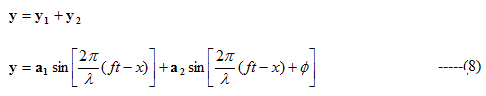Now from trigonometry identityPutting it in equation (8) we find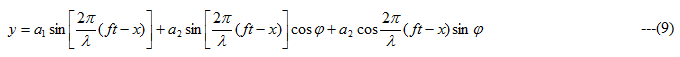Let us suppose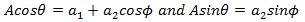Putting them in equation (9) we havewhere• We know that intensity of waves is proportional to its amplitude i.e.